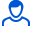Content: 00090.png (58.17 KB)

Positive responses: 0
Negative responses: 0

Sold: 2
Refunds: 0Loyalty discount! If the total amount of your purchases from the seller more than:

 \$1 the discount is 10% \$5 the discount is 30%

Two material points move along the x-axis according to the equations x1 = A1 B1 C1t2 and x2 = A2 B2 C2t2, where A1 = 10 m, B1 = 2 m / s, C1 = -2 m / s2, A2 = 3 m, B2 = 1 m / s, C2 = 0.2 m / s2. Determine the speeds V1 and V2 and the accelerations of these points at the time when their speeds are the same; at the moment in time when the points occupy the same position on the x-axis.
Task 00090. Detailed solution with a brief record of the conditions, formulas and laws used in the decision, the conclusion of the calculation formula and the answer.
If you have any questions about the decision, write. I will try to help. File in image format.
No feedback yet